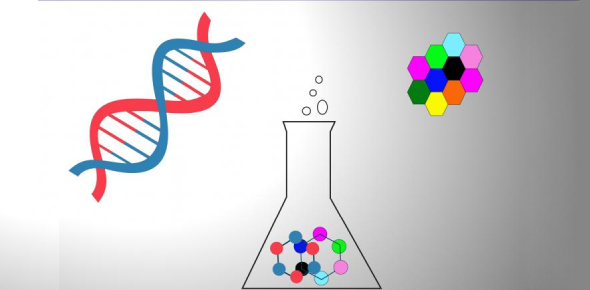# Biochemistry MCQ Quiz: Exam!

15 Questions | Total Attempts: 1790Settings.

• 1.
In a water molecule, hydrogens are partially _____;  oxygens are partially _____.
• A.

Negative; negative

• B.

Negative; positive

• C.

positive; negative

• D.

Positive; positive

• 2.
Hydrogen bonds are approximately _____% of the bond strength of covalent C-C or C-H bonds.
• A.

1

• B.

5

• C.

20

• D.

50

• 3.
Hydrogen bonds within liquid water are _________.
• A.

attractions between the protons of the oxygen nuclei

• B.

Ion-induced dipole interactions

• C.

Attractions between the H+ and OH ions of the liquid

• D.

Dipole-dipole interactions

• 4.
A molecule that has both a polar and nonpolar region is called _____________.
• A.

Micelleic

• B.

Amphiphilic

• C.

Endergonic

• D.

Membrane

• 5.
In aqueous solution, globules of up to several thousand amphiphilic molecules arranged with the hydrophilic groups on the surface and the hydrophobic groups buried in the center are called _____.
• A.

Micelles

• B.

Vacuoles

• C.

Liposomes

• D.

Bilayers

• 6.
In an aqueous solution, if the [OH] is 3.0´10-5 M, what is the [H+]?
• A.

7.0´10-9

• B.

7.0´10-2

• C.

3.3´10-10

• D.

3.3´10-3

• 7.
What would be the resulting pH if one drop (0.05 ml) of 1.0 M HCl was added to one liter of pure water (assume pH 7.0)?
• A.

2.7

• B.

4.3

• C.

5.0

• D.

0 (there would be no significant change)

• 8.
Which of the following could be used to formulate 100 mls of a 0.10 M phosphate buffer (pK=6.82) at pH 7.2?
• A.

2.9 mmoles of Na2HPO4 and 7.1 mmoles of NaHPO4

• B.

10 mmoles of Na2HPO4 and 7.1 mmoles of NaOH

• C.

10 mmoles of NaHPO4 and 7.1 mmoles of HCl

• D.

All of the above

• 9.
What is the resulting pH if 10 millimoles of HCl is added to 1 liter of a 0.1 M phosphate buffer at pH 7.00 (pK=6.82)?
• A.

6.82

• B.

6.98

• C.

7.01

• D.

7.19

• 10.
At any given moment, a single water molecule participates in _____ strong hydrogen bond(s).  The role played by the water molecule is best characterized as _____.
• A.

two ; one H-bond donor, one H-bond acceptor

• B.

Two ; two H-bond donor

• C.

Two ; two H-bond acceptor

• D.

One; H-bond donor

• 11.
In a hydrogen bond between a water molecule and another biomolecule, _____
• A.

A hydrogen ion on the water molecule forms an ionic bond with a hydride ion on the other molecule

• B.

the hydrogen bond will typically form between a hydrogen atom and either a nitrogen, sulfur, or oxygen atom

• C.

The partial charge on a hydrogen of the water interacts with the partial charge on a hydrogen of the other molecule

• D.

A hydrogen on the water molecule forms a covalent bond to an oxygen or nitrogen atom on the other molecule

• 12.
The strongest non-covalent interactions are _____
• A.

Van der Waals interactions

• B.

London dispersion forces

• C.

Hydrogen bonds

• D.

Ionic interactions

• 13.
Due to the formation of hydrogen bonds, _____ is highly soluble in water.
• A.

carbon dioxide

• B.

Sodium chloride

• C.

Methanol

• D.

Octane

• 14.
Which of the following functional groups has two hydrogen bond donors and one hydrogen bond acceptor?
• A.

alcohol

• B.

Ester

• C.

Thiol

• D.

Amine

• 15.
What term is used to describe the exclusion of nonpolar substances from an aqueous solution?
• A.

Nonpolar effect

• B.

Lipid effect

• C.

hydrophobic effect

• D.

Oil droplet effect

Related TopicsBack to top